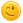# Write a program that checks whether an integer number is divisible by 2 and 3, by 2 or 3, and by 2 or 3 but not both.

+1 vote
Write a program that checks whether an integer number is divisible by 2 and 3, by 2 or 3, and by 2 or 3 but not both.

answered Mar 25, 2019 by Nitin Rauthan
#include <stdio.h>

int check_div(int n)
{

if(n%2==0)
{
if(n%3==0)
{
printf("Divisible by 2 and 3 both\n");
return 1;
}
printf("Divisible by 2 only\n");
return 2;
}
if(n%3==0)
{
printf("Divisible by 3 only\n");
return 3;
}

printf("Neither Divisible by 2 nor 3\n");
return 0;

}

int main()
{
int n;

printf("Enter the number\n");

scanf("%d",&n);

check_div(n);

return 0;
}
answered Jul 30, 2019 by anonymous
#include<stdio.h>

int main(){

int a;

scanf("%d",&a);

if(a%6==0)

printf("divisible by both 2 and 3");

else if(a%2==0)

printf("divisible by 2");

else if (a%3==0)

printf("divisible by 3");

else

printf("divisible by neither 2 nor 3");

return 0;

}
answered Jul 31, 2019 by (300 points)
#include<stdio.h>
int main()
{
int n;
scanf("%d",&n);
if(n%6==0)
{
if(n%3==0&&n%2==0)
printf("divisible by both 3 and 2");
else if(n%3==0)
printf("divisible by 3");
else if(n%2==0)
printf("divisible by 2");
else if(n%2!=0&&n%3!=0)
printf("neither 2 nor 3");
}
else
printf("no");
return 0;
}
Try this one .